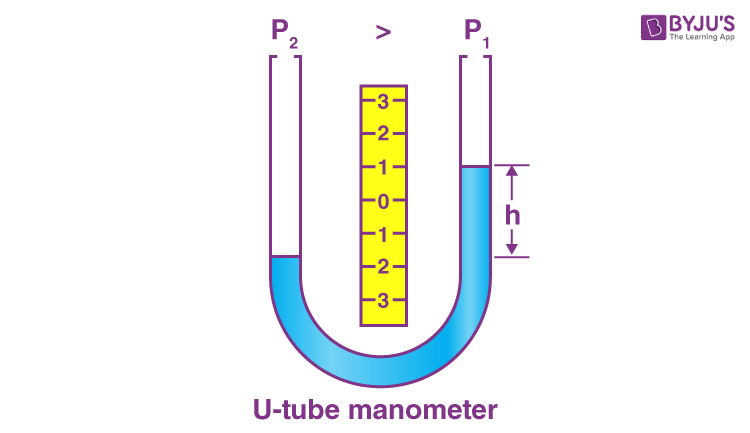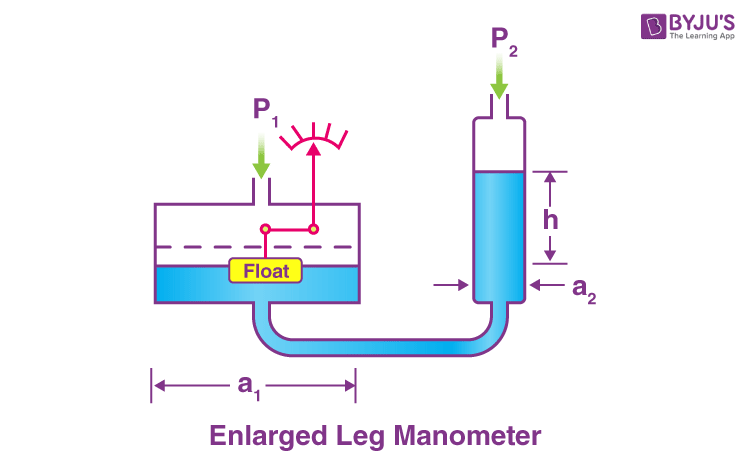Checkout JEE MAINS 2022 Question Paper Analysis : Checkout JEE MAINS 2022 Question Paper Analysis :

# Manometer

We know there are different kinds of pressure around us. Pressure in simple words is described as the physical force exerted on an object. We know that atmospheric pressure is measured using an instrument called a barometer. In this article, let us learn how fluid pressure is measured with respect to Earth’s atmosphere using a device known as a manometer.

Fluid pressure is given by the following equation: P = ρgh

Where,

P is the fluid pressure

ρ is the density of the fluid

g is the acceleration due to gravity

h is the depth

## Manometer and Classification Of Manometers

Manometer is a device that measures the fluid pressure. Based on various criteria, manometers are classified into different types. They are:

1. U-tube Manometer
2. Enlarged Leg Manometer
3. Well Type Manometer
4. Inclined Tube Manometer

### U-Tube Manometer

U-tube manometer features a vertical or inclined U-tube column that is filled with a reference liquid (mercury) to display the pressure level. When the columns of the device are exposed to the atmosphere, the level of liquid in the limbs are equal and this indicates the atmospheric pressure. When one of the columns is connected to the pressure vessel, there will be a difference in the level of the liquid in the limbs, which signifies the pressure of the liquid in the vessel.

A simple U-tube manometer is as shown in the figure below. This type of manometer includes nill moving parts and requires no calibration.From the figure above we can see that, when the pressure is applied to the left side of a U-tube manometer, the left leg shows the lowering of liquid and rises in the right leg. The liquid moves until the unit weight of the liquid balances the pressure. It is represented by the letter h.

### Enlarged Leg ManometerIn this type of manometer, float is seen in the enlarged leg that is used for noting or indicating. In an enlarged leg manometer two legs can be changed for changing the measurement span.

### Well Type Manometer

A well type manometer includes a large well in one of the legs. Another leg features a simple tube. A minute change in the displacement of the liquid level in the well, there shall be the large change in the height of the tube. U-tube manometers can be used in place of a large well, such that the variation in the level in the well will be negligible and instead of measuring a differential height, a single height in the remaining column is measured.

The area of the well is much greater than the area of the tube. In general, this ratio is greater than 10.

### Inclined Tube Manometer

It is a slant shaped manometer which has a 10 degree angle of measuring leg. The sensitivity of the device is improved with the inclination. This manometer is used to measure the small pressure differences.

Stay tuned with BYJU’S for more such interesting articles. Also, register to “BYJU’S – The Learning App” for loads of interactive, engaging Physics-related videos.

## Frequently Asked Questions on Manometer

P = ρgh

### 2. What is a manometer?

A manometer is used for measuring the fluid pressure with respect to an outside source which is usually considered to be the earth’s atmosphere.

Mercury.

### 4. What are the different types of manometers?

• U-tube Manometer
• Enlarged Leg Manometer
• Well Type Manometer
• Inclined Tube Manometer

10 degrees.